# Angle Sum Property Of A Triangle & Exterior Angle Theorem

A triangle is the smallest polygon which has three sides and three interior angles. In this article, we are going to discuss the angle sum property and the exterior angle theorem of a triangle with its statement and proof in detail.

## Angle Sum Property of a Triangle Theorem

In the given triangle, ∆ABC, AB, BC, and CA represent three sides. A, B and C are the three vertices and ∠ABC, ∠BCA and ∠CAB are three interior angles of ∆ABC.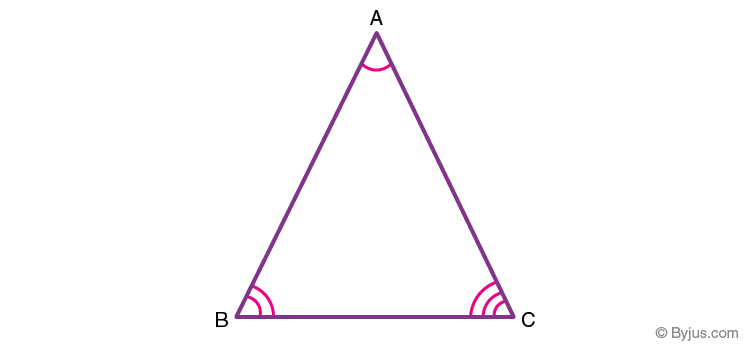Theorem 1: Angle sum property of triangle states that the sum of interior angles of a triangle is 180°.

Proof:

Consider a ∆ABC, as shown in the figure below. To prove the above property of triangles, draw a line $\overleftrightarrow {PQ}$ parallel to the side BC of the given triangle.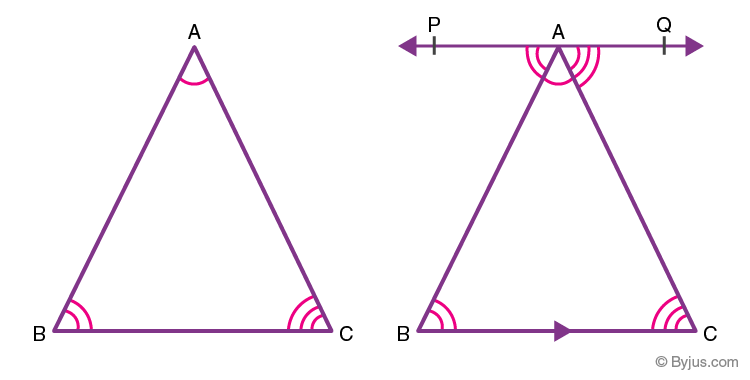Since PQ is a straight line, it can be concluded that:

∠PAB + ∠BAC + ∠QAC = 180°  ………(1)

Since PQ||BC and AB, AC are transversals,

Therefore, ∠QAC = ∠ACB (a pair of alternate angle)

Also, ∠PAB = ∠CBA (a pair of alternate angle)

Substituting the value of ∠QAC and∠PAB in equation (1),

∠ACB + ∠BAC + ∠CBA= 180°

Thus, the sum of the interior angles of a triangle is 180°.

## Exterior Angle Property of a Triangle Theorem

Theorem 2: If any side of a triangle is extended, then the exterior angle so formed is the sum of the two opposite interior angles of the triangle.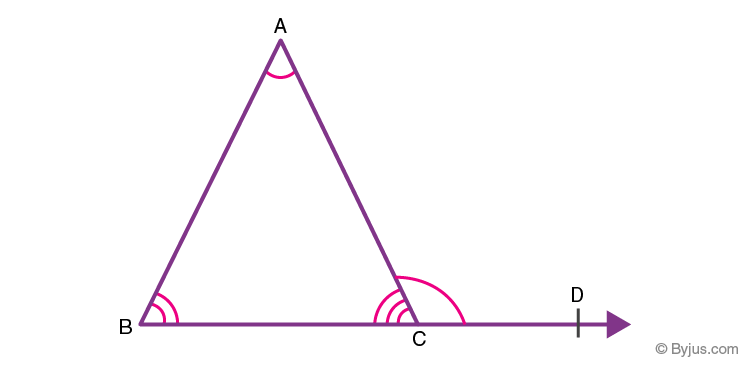In the given figure, the side BC of ∆ABC is extended. The exterior angle ∠ACD so formed is the sum of measures of ∠ABC and ∠CAB.

Proof:

From figure 3, ∠ACB and ∠ACD form a linear pair since they represent the adjacent angles on a straight line.

Thus, ∠ACB + ∠ACD = 180°  ……….(2)

Also, from the angle sum property, it follows that:

∠ACB + ∠BAC + ∠CBA = 180° ……….(3)

From equation (2) and (3) it follows that:

∠ACD = ∠BAC + ∠CBA

This property can also be proved using the concept of parallel lines as follows: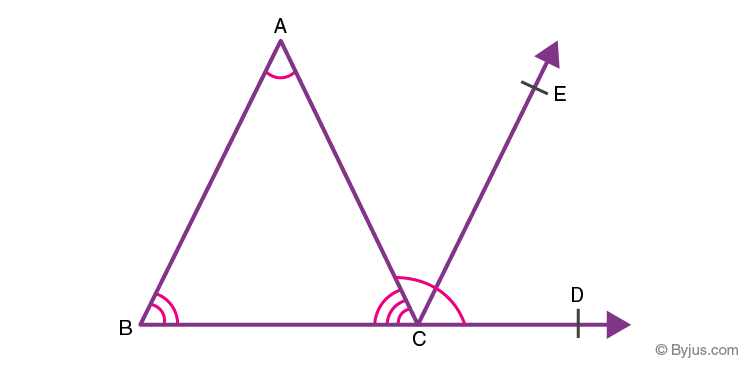In the given figure, side BC of ∆ABC is extended. A line $\overleftrightarrow {CE}$ parallel to the side AB is drawn, then: Since $\overline {BA} ~||~\overline{CE}$ and $\overline{AC}$ is the transversal,

∠CAB = ∠ACE   ………(4) (Pair of alternate angles)

Also, $\overline {BA} ~||~\overline{CE}$ and $\overline{BD}$ is the transversal

Therefore, ∠ABC = ∠ECD  ……….(5) (Corresponding angles)

We have, ∠ACB + ∠BAC + ∠CBA = 180° ………(6)

Since the sum of angles on a straight line is 180°

Therefore, ∠ACB + ∠ACE + ∠ECD = 180° ………(7)

Since, ∠ACE + ∠ECD = ∠ACD(From figure 4)

Substituting this value in equation (7);

∠ACB + ∠ACD = 180° ………(8)

From the equations (6) and (8) it follows that,

∠ACD = ∠BAC + ∠CBA

Hence, it can be seen that the exterior angle of a triangle equals the sum of its opposite interior angles.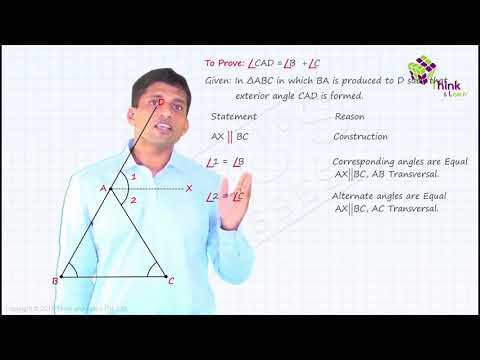Test your Knowledge on Angle Sum Property

1. Vedika Kumar

I alerdy have tablet of byjus premium.

2. JIYA CHHAJER

loved it explaination was so clearly explained which drew my mind towards it also it helped me to gain knowledge ,hoping to book a byjus class soon ,NICE EXPERIENCE, VERY HELPFUL .

3. Aaron Samson

Good Going byju’s anyways thanks for the information.
User of Byju’s app

4. Shresth Bhatia

Thanks for the video really helpfull, cleared my doubts
User of Byus App

5. Aniyah Berger